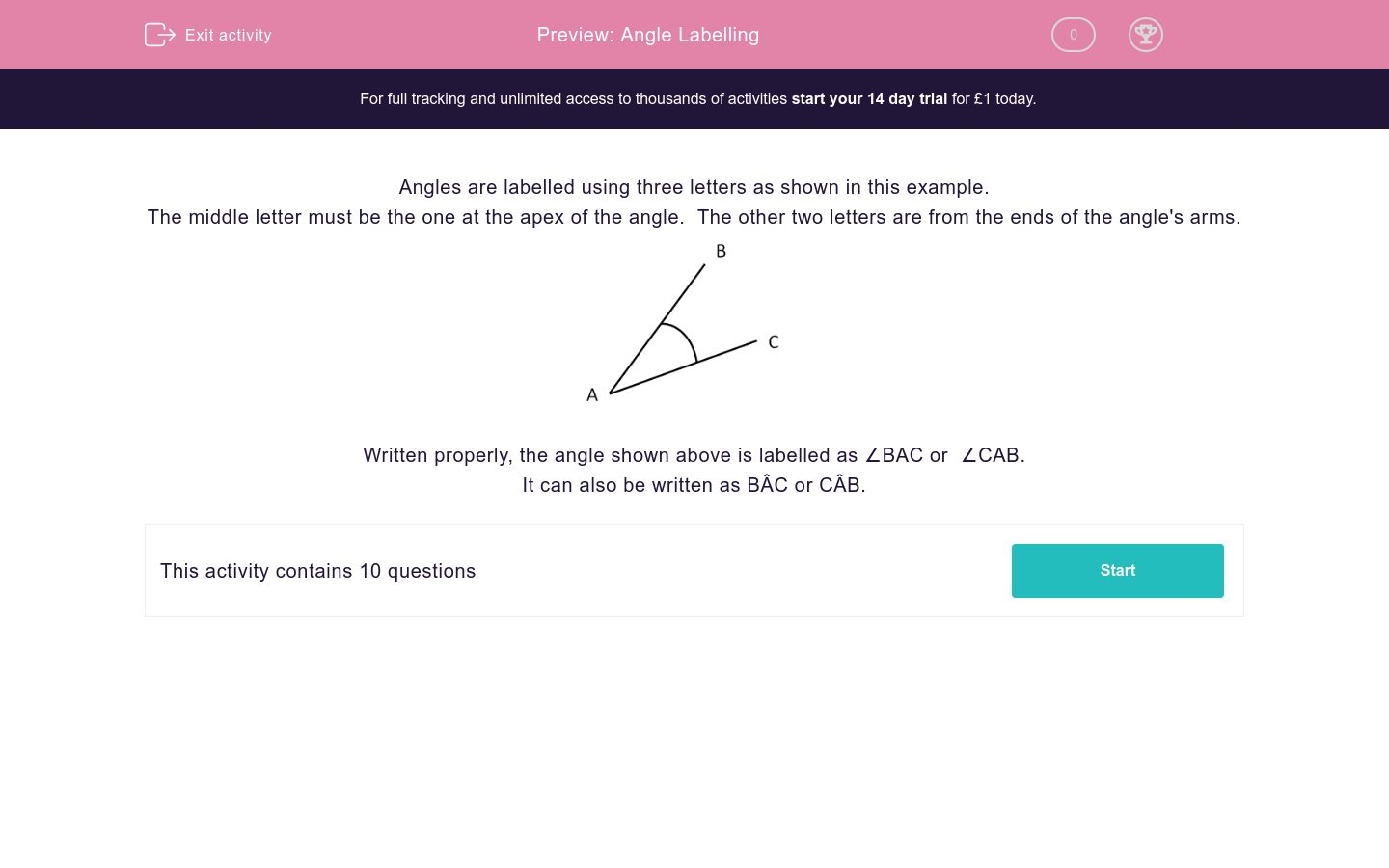# Angle Labelling

In this worksheet, students label angles using the correct three letters.Key stage:  KS 2

Curriculum topic:   Maths and Numerical Reasoning

Curriculum subtopic:   2D Shapes: Circles, Angles and Bearings

Difficulty level:\$appendString = '&new=1'; \$_SESSION['guest_aId'] = rand(); \$urlToRedirect = FRAMEWORK_PATH . 'preview/worksheet/introduction?aId=' . \$_SESSION['guest_aId'] . '&wId=' . \$wsData['id'] . '&usertype=guest' . \$appendString; ?>

### QUESTION 1 of 10

Angles are labelled using three letters as shown in this example.

The middle letter must be the one at the apex of the angle.  The other two letters are from the ends of the angle's arms.Written properly, the angle shown above is labelled as ∠BAC or  ∠CAB.

It can also be written as BÂC or CÂB.

Select all the ways of correctly labelling the angle shown in the diagram.∠ECB

∠EBC

∠BEC

∠BCE

∠CBE

∠CEB

Select all the ways of correctly labelling the angle shown in the diagram.∠ECD

ÊCD

∠DEC

∠DCE

CÊD

∠CED

Select all the ways of correctly labelling the angle shown in the diagram.∠CFG

CFG

∠FGC

∠CGF

∠GFC

∠F

Select all the ways of correctly labelling the angle shown in the diagram.∠FPH

PFH

∠PFH

∠PHF

∠HFP

∠F

Select all the ways of correctly labelling the angle shown in the diagram.∠ROP

POR

∠POR

PÔR

∠QOP

RÔP

Select all the ways of correctly labelling the angle shown in the diagram.∠RPI

PIR

∠PIQ

PÎQ

∠QIP

RÎQ

Select all the ways of correctly labelling the angle shown in the diagram.∠RPI

Q

∠RIQ

PÎQ

∠IQR

RÎQ

Select all the ways of correctly labelling the angle shown in the diagram.∠PAH

∠TAP

∠HAT

TÂH

∠ATH

PÂT

Select all the ways of correctly labelling the angle shown in the diagram.∠PAH

∠TAP

∠HAT

TÂH

∠ATH

PÂT

Select all the ways of correctly labelling the angle shown in the diagram.∠JIH

∠HIJ

∠JIF

JÎH

∠JKI

FÎJ

• Question 1

Select all the ways of correctly labelling the angle shown in the diagram.∠BEC
∠CEB
EDDIE SAYS
If we start at B, we go from B to E to C, therefore the angle is ∠BEC It can also be the other way round: ∠CEB
• Question 2

Select all the ways of correctly labelling the angle shown in the diagram.∠DEC
CÊD
∠CED
EDDIE SAYS
If we start at C, we go from C to E to D, therefore the angle is ∠CED It can also be the other way round: ∠DEC It can (but isn\'t very often, written with an angle over the middle letter. CÊD or DÊC
• Question 3

Select all the ways of correctly labelling the angle shown in the diagram.∠CFG
∠GFC
EDDIE SAYS
If we start at C, we go from C to F to G, therefore the angle is ∠CFG It can also be the other way round: ∠GFC
• Question 4

Select all the ways of correctly labelling the angle shown in the diagram.∠PFH
∠HFP
EDDIE SAYS
If we start at P, we go from P to F to H, therefore the angle is ∠PFH It can also be the other way round: ∠HFP
• Question 5

Select all the ways of correctly labelling the angle shown in the diagram.∠ROP
∠POR
PÔR
RÔP
EDDIE SAYS
If we start at R, we go from R to O to P, therefore the angle is ∠ROP It can also be the other way round: ∠POR It can (but isn\'t very often, written with an angle over the middle letter. PÔR or RÔP
• Question 6

Select all the ways of correctly labelling the angle shown in the diagram.∠PIQ
PÎQ
∠QIP
EDDIE SAYS
If we start at P, we go from P to I to Q, therefore the angle is ∠PIQ It can also be the other way round: ∠QIP It can (but isn\'t very often, written with an angle over the middle letter. PÎQ or QÎP
• Question 7

Select all the ways of correctly labelling the angle shown in the diagram.∠IQR
EDDIE SAYS
If we start at R, we go from R to Q to I, therefore the angle is ∠RQI It can also be the other way round: ∠IQR
• Question 8

Select all the ways of correctly labelling the angle shown in the diagram.∠HAT
TÂH
EDDIE SAYS
If we start at T we go from T to A to H, therefore the angle is ∠TAH It can also be the other way round: ∠HAT It can (but isn\'t very often, written with an angle over the middle letter. TÂH or HÂT
• Question 9

Select all the ways of correctly labelling the angle shown in the diagram.∠TAP
PÂT
EDDIE SAYS
If we start at T we go from T to A to P, therefore the angle is ∠TAP It can also be the other way round: ∠PAT It can (but isn\'t very often, written with an angle over the middle letter. TÂP or PÂT
• Question 10

Select all the ways of correctly labelling the angle shown in the diagram.∠JIF
FÎJ
EDDIE SAYS
If we start at F we go from F to I to J, therefore the angle is ∠FIJ It can also be the other way round: ∠JIF It can (but isn\'t very often, written with an angle over the middle letter. JÎF or FÎJ
---- OR ----

Sign up for a £1 trial so you can track and measure your child's progress on this activity.

### What is EdPlace?

We're your National Curriculum aligned online education content provider helping each child succeed in English, maths and science from year 1 to GCSE. With an EdPlace account you’ll be able to track and measure progress, helping each child achieve their best. We build confidence and attainment by personalising each child’s learning at a level that suits them.

Get started